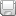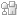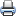Home > Articles > Cisco Certification > CCNA Routing and Switching > Cisco CCENT Practice and Study Guide: Subnetting IP Networks

# Cisco CCENT Practice and Study Guide: Subnetting IP NetworksSaveDiggDel.icio.usPrint

### Contents

1. Subnetting an IPv4 Network
3. Design Considerations for IPv6

### Chapter Description

This chapter reviews the process of subnetting IP networks. First, by reviewing a process for subnetting IPv4 networks, then, by practicing subnetting skills, including several scenarios.

### From the Book\$25.50 (Save 15%)

Understanding the hierarchical structure of the IP address and how to modify that hierarchy in order to more efficiently meet routing requirements is an important part of planning an IP addressing scheme. This chapter reviews the process of subnetting IP networks. First, we review a process for subnetting IPv4 networks. Then, you practice subnetting skills, including several scenarios. Then, we briefly review subnetting IPv6 networks.

## Subnetting an IPv4 Network

The process of segmenting a network, by dividing it into multiple smaller network spaces, is called subnetting. These subnetworks are called subnets. Although subnetting calculators are plentiful and freely accessible on the Internet, you must know how to subnet without using a calculator when you sit for the CCENT exam. Furthermore, subnetting skill will serve you well when troubleshooting common IP addressing issues.

### Subnetting in Four Steps

Everyone has a preferred method of subnetting. Each teacher will use a slightly different strategy to help students master this crucial skill. The method I prefer can be broken down into four steps:

Step 1. Determine how many bits to borrow based on the network requirements.

Step 2. Determine the new subnet mask.

Step 3. Determine the subnet multiplier.

### Subnetting Example

The best way to demonstrate the four steps of subnetting is to use an example. Let’s assume that you are given the network address 192.168.1.0/24, you need 30 hosts per network, and want to create as many subnets as possible.

#### Determine How Many Bits to Borrow

Because our requirement specifies 30 host addresses per subnet, we need to first determine the minimum number of host bits to leave. The remaining bits can be borrowed:

Host Bits = Bits Borrowed + Bits Left

To provide enough address space for 30 hosts, we need to leave 5 bits. Use the following formula:

2BL – 2 = number of host addresses

where the exponent BL is bits left in the host portion.

Remember, the “minus 2” is to account for the network and broadcast addresses that cannot be assigned to hosts.

In this example, leaving 5 bits in the host portion will provide the right number of host address:

25 – 2 = 30

Because we have 3 bits remaining in the original host portion, we borrow all these bits to satisfy the requirement to “create as many subnets as possible.” To determine how many subnets we can create, use the following formula:

2BB = Number of subnets

where the exponent BB is bits borrowed from the host portion.

In this example, borrowing 3 bits from the host portion will create 8 subnets: 23 = 8.

As shown in Table 9-1, the 3 bits are borrowed from the far-left bits in the host portion. The highlighted bits in the table show all possible combinations of manipulating the 8 bits borrowed to create the subnets.

#### Table 9-1 Binary and Decimal Value of the Subnetted Octet

 Subnet Number Last Octet Binary Value Last Octet Decimal Value 0 00000000 .0 1 00100000 .32 2 01000000 .64 3 01100000 .96 4 10000000 .128 5 10100000 .160 6 11000000 .192 7 11100000 .224

#### Determine the New Subnet Mask

Notice in Table 9-1 that the network bits now include the 3 borrowed host bits in the last octet. Add these 3 bits to the 24 bits in the original subnet mask and you have a new subnet mask, /27. In decimal format, you turn on the 128, 64, and 32 bits in the last octet for a value of 224. So, the new subnet mask is 255.255.255.224.

#### Determine the Subnet Multiplier

Notice in Table 9-1 that the last octet decimal value increments by 32 with each subnet number. The number 32 is the subnet multiplier. You can quickly find the subnet multiplier using one of two methods:

• Method 1: Subtract the last nonzero octet of the subnet mask from 256. In this example, the last nonzero octet is 224. So, the subnet multiplier is 256 – 224 = 32.
• Method 2: The decimal value of the last bit borrowed is the subnet multiplier. In this example, we borrowed the 128 bit, the 64 bit, and the 32 bit. The 32 bit is the last bit we borrowed and is, therefore, the subnet multiplier.

By using the subnet multiplier, you no longer have to convert binary subnet bits to decimal.

Listing the subnets, host ranges, and broadcast addresses helps you see the flow of addresses within one address space. Table 9-2 documents our subnet addressing scheme for the 192.168.1.0/24 address space. Fill in any missing information.

#### Table 9-2 Subnet Addressing Scheme for 192.168.1.0/24: 30 Hosts Per Subnet

 Subnet Number Subnet Address Host Range Broadcast Address 0 192.168.1.0 192.168.1.1–192.168.1.30 192.168.1.31 1 192.168.1.32 192.168.1.33–192.168.1.62 2 192.168.1.65–192.168.1.94 192.168.1.95 3 192.168.1.96 4 192.168.1.128 192.168.1.129–192.168.1.158 192.168.1.159 5 192.168.1.161–192.168.1.190 192.168.1.191 6 192.168.1.192 7

Use the four subnetting steps to complete the following scenarios.

### Subnetting Scenario 1

Subnet the address space 10.10.0.0/16 to provide at least 100 host addresses per subnet while creating as many subnets as possible.

1. How many bits should your borrow?
2. What is the new subnet mask in dotted-decimal and prefix notation?

3. What is the subnet multiplier?

In Table 9-3, list the first three subnets, host ranges, and broadcast addresses.

### Subnetting Scenario 2

Subnet the address space 10.10.0.0/16 to provide at least 500 subnet addresses.

1. How many bits should your borrow?
2. What is the new subnet mask in dotted-decimal and prefix notation?

3. What is the subnet multiplier?

In Table 9-4, list the first three subnets, host ranges, and broadcast addresses.

### Subnetting Scenario 3

Subnet the address space 10.10.10.0/23 to provide at least 60 host addresses per subnet while creating as many subnets as possible.

1. How many bits should your borrow?
2. What is the new subnet mask in dotted-decimal and prefix notation?

3. What is the subnet multiplier?

In Table 9-5, list the first three subnets, host ranges, and broadcast addresses.